## Example Questions

### Example Question #3 : New Sat Math No Calculator

If a polygon has 10 sides, what is the measure of each exterior angle?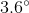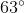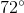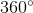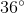Explanation:

In order to figure this out, we need to remember the formula for finding exterior angles.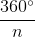, whereis the number of sides of a polygon. Now we simply do the following calculation.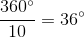So the measure of each exterior angle is.